1. 预测图像旋转方向
2. 预测图片补丁位置相对关系
3. 补丁拼图
4. 图片上色
5. 自编码器系列
6. 对抗神经网络
7. 对比学习

1. 样本Pair对如何构造
2. Loss 如何设计
1. Contrast Loss
2. Triplet Loss
3. N-Pair Loss
4. InfoNCE Loss

1. 正负Pair自监督对比学习
1. SimCLR
2. MOCO系列
2. 聚类自监督对比学习
1. SmAV
3. 非对称结构的自监督学习
1. SimSiam
4. 对比学习模型总结

1. SimSiam实现
2. SimCLR 实现
3. 实现细节
1. 自监督+finetune训练过程
2. 监督训练过程
3. 结果评测

1.监督学习。训练数据有标注（人工标注出准确的标签），比如典型的图像分类，给一张图片打上标签（类别），算法来通过学习来预测类别从而得到预测规则（模型参数）。目前机器学习取得较大突破的基本都是这类，而且取的进展比较大领域一般都是可以低成本的获取标注数据（比如推荐系统等）。

2.无监督学习。没有明确目标下发现数据的规律，比如最常见的聚类、降维等。

4.强化学习。智能体通过观察和接受环境的信息，寻求并学习找到奖励最大化的行动，整个反馈在整个运行过程中收集，比如在围棋上成名的AlphaGo。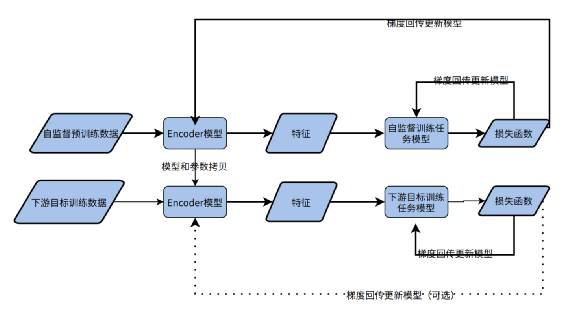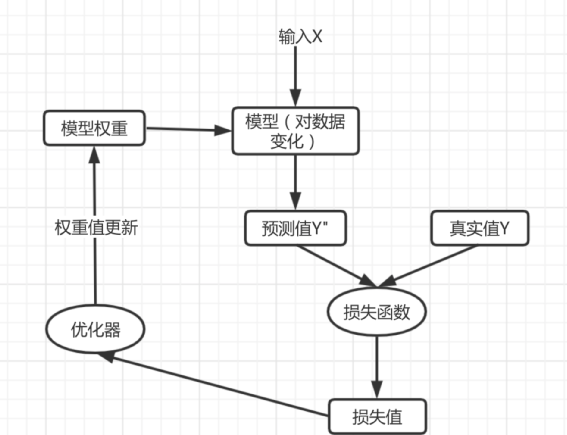1.预测图像旋转方向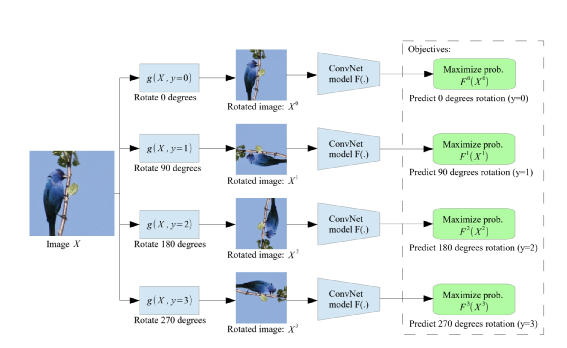1. 原始图像会经过 0、90、180、270 四个角度方向旋转
2. 然后Encoder输出的特征用来进行4分类判别

2.预测图片补丁位置相对关系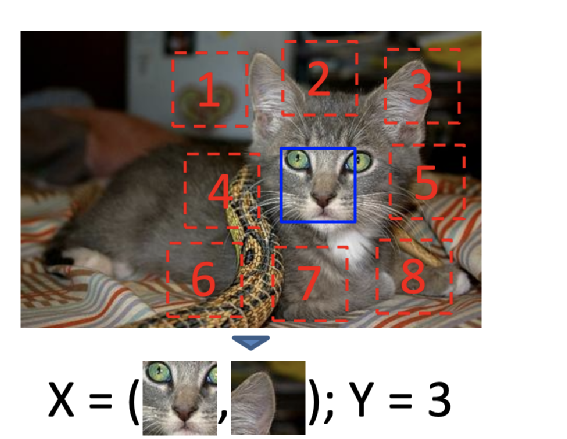1. 在图像上随机裁剪得到正方形图像；
2. 对正方形图像裁剪成9个大小小相等正方形区域（注：裁剪过程有一些增强技巧如小图片增加缝隙、抖动增强详见附录论文2）；
3. 选取9个小图的中心小图和除外一个随机小图，这两个图片经过Encoder后特征拼接进入全连接分类图，分类类别为8。
3.补丁拼图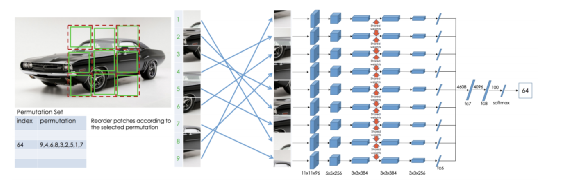1. 在图像上随机裁剪得到正方形图像；
2. 并对正方形图像裁剪成9个大小小相等正方形区域，同预测补丁相对位置关系，裁剪图片也有一些技巧，见论文3；
3. 将9个小图随机打散顺序得到一种基于原图顺序排列组合，然后每个小图进入Encoder得到特征输出，特征进入各自的全连接网络（不共享）后输出变换特征
4. 变换特征拼接后进入MLP得到分类类别，理论上一种排列组合可以认为是一种类别，所有类别总数为：9！=362880。作者认为这么多类别中有很多很相似没法训练模型提取有效的特征，所以通过序列字符串汉明距离来选择差异很大的序列作为类别。
4.图片上色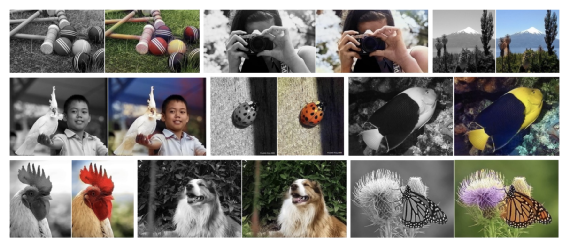1. 灰度图经过Encoder得到 特征；
2. 特征经过Decoder重建得到上色图片；
3. 上色图片和原图计算Loss，常见的上色任务是计算上色后图片与原图的L1或者L2 Loss，在附图论文中Loss做了一些特殊处理：颜色模式不是RGB而是LAB（参考4），此外将AB 颜色通道量化为313个区间，最终目标是求每个像素点在313区间的分类概率，根据将分类概率Top N 平滑后选择上色。
5.自编码器系列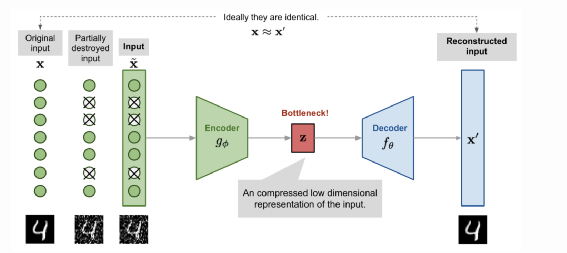1. 原始图像经过扰动加入噪点
2. 噪点图像经过Encoder编码后得到特征Z
3. 特征Z经过Decoder得到重建图片，要求重建图像和原始图像尽可能一致
6.对抗神经网络

7.对比学习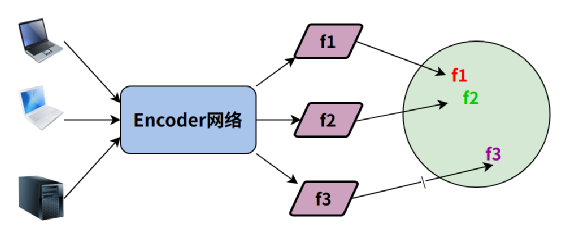1.样本Pair对如何构造

• 随机裁剪
• 随机噪音
• 高斯模糊
• 抖动
• 颜色通道转化
• 灰度化
• 对比度调节
• 亮度调节
2.Loss 如何设计

1.Contrast Loss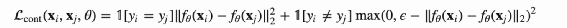ϵ是超参数，希望的是负样本对之间L2的距离应该大于它。当正样本对的学习的特征一致（L2距离等于0）、负样本对之间特征L2 大于ϵ时，Loss为0，只要最小化Loss既可。
2.Triplet Loss
Triplet Loss是深度度量学习中比较常用的Loss，效果一般优化Contrast Loss，公式如下：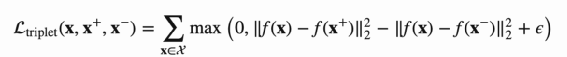ϵ是超参数，Triplet Loss 以f(x)作为”锚点“。希望最小化正样本对距离同时最大化负样本对之间的距离，且负样本对距离相比正样本对的距离要超过ϵ，原理如下图：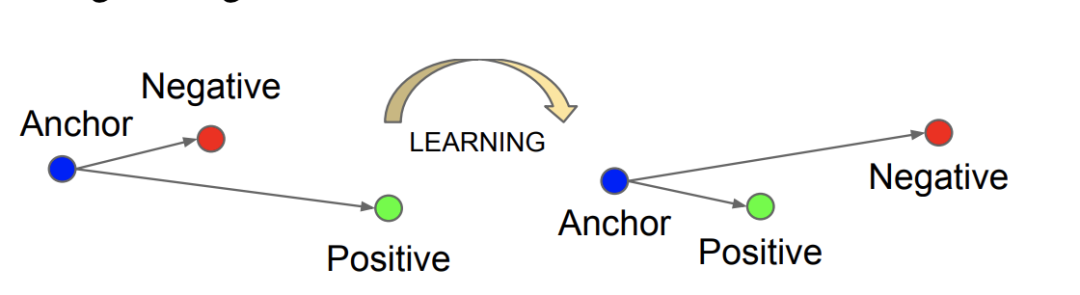3.N-Pair Loss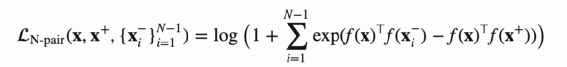4.InfoNCE Loss
InfoNCE Loss 思想取决于NCE，NCE希望是通过概率预估，利用逻辑回归可以区分数据中的正样本和噪音数据，而InfoNCE Loss来自CPC（Contrastive Predictive Coding）预估，可以是过分类交叉熵来区分正样本和噪音数据，公式如下：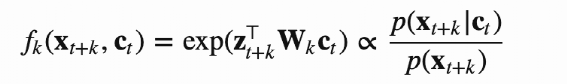1.正负Pair自监督对比学习

1.SimCLR
SimCLR 原理如下图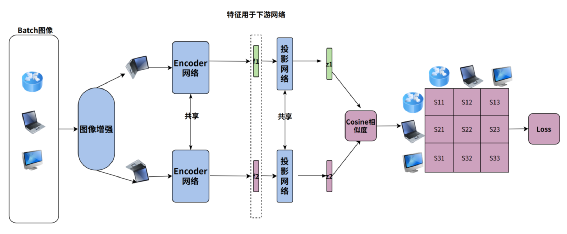2.MOCO系列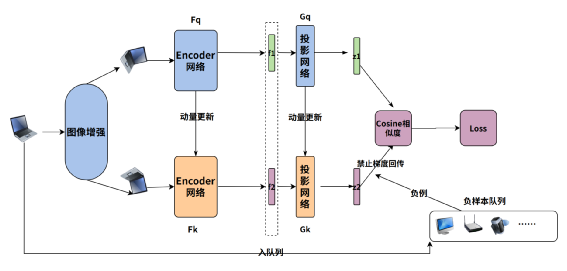• 负样本通过定长队列维护保存，每个Batch淘汰老数据，加入新数据

• 正例网络（Fq,Gq）用标准的梯度下降优化，负例网络（Fk,Gk）采用正例的模型参数的动量更新，即：Fk <- Fk*m+(1-m)*Fq，Gk <- Gk*m+(1-m)*Gq，代码如下：

``@torch.no_grad()``def _momentum_update_key_encoder(self):``"""``    Momentum update of the key encoder``    """``for param_q, param_k in zip(self.encoder_q.parameters(), self.encoder_k.parameters()):``        param_k.data = param_k.data * self.m + param_q.data * (1. - self.m)``

2.聚类自监督对比学习

1.SmAV
2020年《Swapping Assignments between multiple Views of the same images》被提出，利用聚类来进行样本区分，目前在一些公开数据集合上效果逼近监督学习，SmAV原理图如下x`: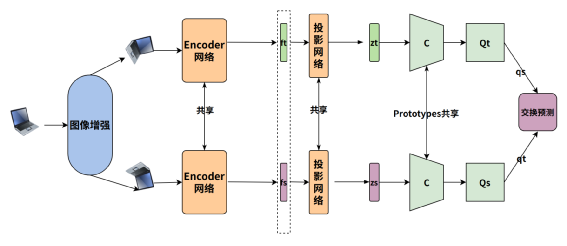1. 两个投影向量分别会和一个KxD 的向量（图中的C论文作者称之Prototypes）相乘得到变换后的向量，这个K可以假设就是K个类，每一行向量可以代表这个类表示（聚类中心），可以认为相乘后的结果就是图片表征向量和K类的相似度，记作为ZtCZsC，其中C是参与学习。
2. 引入了 Q=[q1,…,qB]∈ℝK×B，可以认为Q就是这B个（Batch Size）图片对应的最理想的聚类中心，论文作者尝试Q分别为离散值和连续值的效果，目前连续值的效果更好。
3. 希望ZC和Q 相似度尽可能高，论文作者采用最优传输的理念也就是将一个分布传输到另一个分布的代价做大，来优化C，论文采用的是Sinkhorn 进行最优传输解，见附录17
4. ZtCZsC分别经过Qt和Qs变化后的得到qs和qt，qs和qt可以认为就是聚类结果的label
5. 然后采用交换预测的方法，也就是t的聚类结果去预测s，同样s也去预测t，loss采用交叉熵，如下：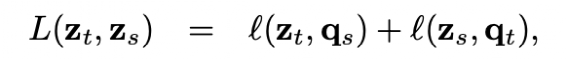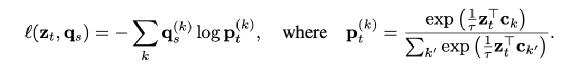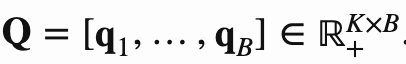Q=[q1,…,qB]∈ℝK×B 满足以下条件：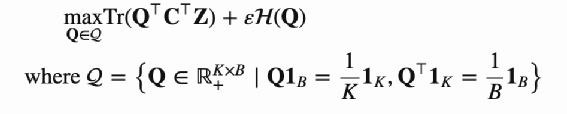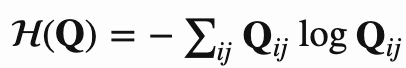3.非对称结构的自监督学习

1.SimSiam
SimSiam 整体结构非常简单，无负样本，原理图如下：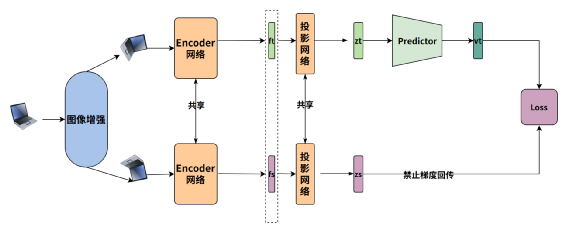1. 一张图像经过两次不同增强后，再经过Encoder编码的图像特征经过投影变化后得到特征Zt，Zs
2. 对于Zt，Zs 再分别一个Predictor网络(MLP）变化得到Vt和Vs（为了避免流程图引起歧义只画了Vt）
3. 通过交换预测来计算Loss，也就是希望Zt和Vs 相似，Zs 和 Vt相似

• 一路的特征Z需要经过Predictor变化，若没有Predictor变化得到V，直接计算两个Z的loss，模型会崩塌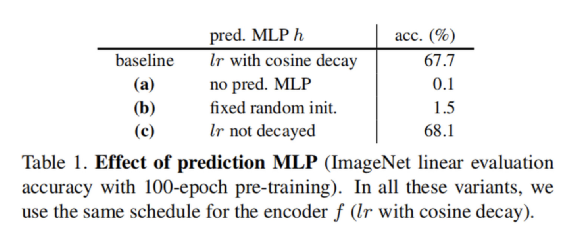• Predictor 的MLP的隐层输出需要进行Batchnorm（但输出层不能加），否则模型性能退化严重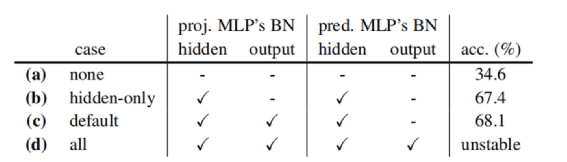• 计算Loss时对于Z要禁止梯度回传，否则模型会崩塌，也就是Loss的计算伪代码如下：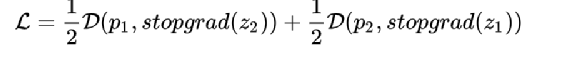4.对比学习模型总结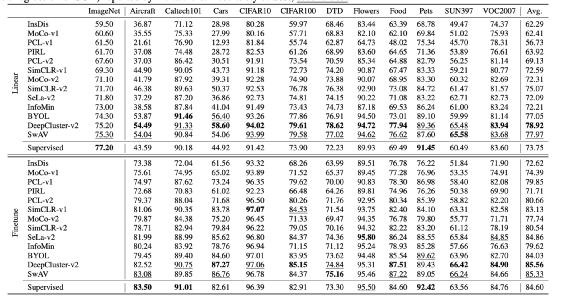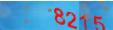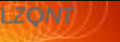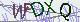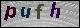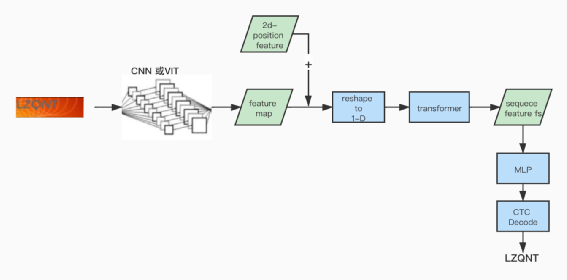1. 因为文字识别不同图像分类，文字识别一张图像里边有多个文字目标，而原始图像识别只有一个目标，如果参考图像分类用fs作为对比特征，很容易出现表征特征混乱，比如两个正样本的特征相似，不能代表每个字符对应的特征相似。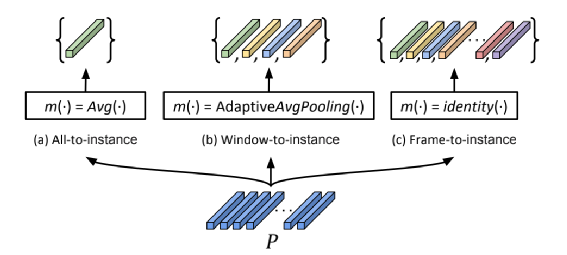``'''``将原始的序列特征 shape 为 B x SeqLen x FeatureDim 的输入特征``转成 shape为 B*ceil(SeqLen/5) x FeatureDim 的输出特征``相当增加Batch Size， 去自监督对比维度为FeatureDim 的特征``'''``def feature_flat(self, feature):``    dim = tf.shape(feature)``    feature = tf.keras.layers.AvgPool1D(pool_size=5, padding="same")(feature)``    feature = tf.reshape(feature, [-1, dim])``return feature``

2. 增强方式的选择，因为人类阅读文本和做图像识别差异，在增强方式不能和原始的图像识别一样，比如左右镜像翻转，文本区域裁剪明显会带来噪音图片，这个自监督任务上，我们选用的增强方式有：
• 随机噪点
• 高斯模糊
• 抖动
• 颜色通道转化
• 灰度化
• 对比度调节
• 亮度调节
• 随机等比缩放图片

1.SimSiam实现

``#预测模型``self.predictor_model = tf.keras.Sequential([``    tf.keras.layers.Dense(``self.LATENT_DIM,``        use_bias=False,``        kernel_regularizer=tf.keras.regularizers.l2(0.001),``    ),``    tf.keras.layers.LeakyReLU(alpha=0.18),``    tf.keras.layers.BatchNormalization(),``    tf.keras.layers.Dense(self.PROJECT_DIM),``]``)``# 投影模型``self.projector_model = tf.keras.Sequential([``    tf.keras.layers.Dense(``self.PROJECT_DIM,``        use_bias=False,``        kernel_regularizer=tf.keras.regularizers.l2(0.001),``    ),``# 模型崩溃，加入batchnorm``    tf.keras.layers.BatchNormalization(),``    tf.keras.layers.LeakyReLU(alpha=0.18),``    tf.keras.layers.Dense(self.PROJECT_DIM),``    tf.keras.layers.BatchNormalization(),``]``)``#simsiam 的前向传播``def forward_sim_siam(self, inputs, training, compute_err, valid):``    parallel = 1``if self.multi_gpu:``        parallel = self.strategy.num_replicas_in_sync``    img = inputs["image"]``    img1, img2 = self.get_aug_img(img, training)``_, _, feature1 = self.model(img1, training=training)``_, _, feature2 = self.model(img2, training=training)``    z1, z2 = self.projector_model(feature1), self.projector_model(feature2)``    p1, p2 = self.predictor_model(z1), self.predictor_model(z2)``    p1, z1, p2, z2 = list(map(self.feature_flat, [p1, z1, p2, z2]))``    loss = self.loss_simsiam(p1, z2) / 2 + self.loss_simsiam(p2, z1) / 2``    result = {"total_loss": loss}``return {k: v / parallel for k, v in result.items()}``#simsiam 的loss计算    ``def loss_simsiam(self, p, z):``'''``     对z要停止梯度回传``    '''``    z = tf.stop_gradient(z)``    p = tf.math.l2_normalize(p, axis=1)``    z = tf.math.l2_normalize(z, axis=1)``'''``    希望的是p和z相似度尽可能大``    '''``return -tf.reduce_mean(tf.reduce_sum((p * z), axis=1))``
2.SimCLR 实现

``# clr 投影模型``self.predictor_model_clr = tf.keras.Sequential([``    tf.keras.layers.Dense(``        self.PROJECT_DIM,``        use_bias=False,``    ),``    tf.keras.layers.LeakyReLU(alpha=0.18),``    tf.keras.layers.Dense(self.PROJECT_DIM),``]``)``def forward_simclr(self, inputs, training, compute_err, valid):``    parallel = 1``    if self.multi_gpu:``        parallel = self.strategy.num_replicas_in_sync``    img1, img2 = self.get_aug_img(inputs["image"], training)``    _, _, feature1 = self.model(img1, training=training)``    _, _, feature2 = self.model(img2, training=training)``    z1, z2 = self.predictor_model_clr(feature1), self.predictor_model_clr(feature2)``    '''``     将sequence feature 转为 frame的feature``    '''``    z1, z2 = self.feature_flat(z1), self.feature_flat(z2)``    loss = self.info_nce_loss(z1, z2)``    result = {"total_loss": loss}``    return {k: v / parallel for k, v in result.items()}`` `` def info_nce_loss(self, projections_1, projections_2):``    projections_1 = tf.math.l2_normalize(projections_1, axis=1)``    projections_2 = tf.math.l2_normalize(projections_2, axis=1)``    similarities = (``            tf.matmul(projections_1, projections_2, transpose_b=True) / 0.1``    )``    batch_size = tf.shape(projections_1)``    contrastive_labels = tf.range(batch_size)``    '''``    希望正样本的图片特征相似度足够大，负样本对相似度足够小，采用交叉熵的方式``    正样本的label=1，负样本=0``    '''``    loss_1_2 = tf.keras.losses.sparse_categorical_crossentropy(``        contrastive_labels, similarities, from_logits=True``    )``    loss_2_1 = tf.keras.losses.sparse_categorical_crossentropy(``        contrastive_labels, tf.transpose(similarities), from_logits=True``    )``    '''``    交叉预测``    '''``    loss = (loss_1_2 + loss_2_1) / 2``    return tf.reduce_mean(loss)``
3.实现细节

1.自监督+finetune训练过程
• 首先SimCLR和SimSiam 采用 SGD+0.8 momentum 优化器 ，lr=3e-4，batch size=96 在80万数据上训练10个epoch
• 接在用ADW优化器，lr=3e-4 ,batch size=8 在4万张标签数据上训练识别模型中MLP（两层全连接网络） 10个epoch
• 最后采用 ADW优化器，lr=3e-5 ,batch size=8 在4万张标签数据上微调识别整个网络 40个epoch
2.监督训练过程
• 直接用ADW 优化器，lr=3e-4 ,batch size=8 在4万张标签数据训练整个识别网络50个epoch
3.结果评测

 监督训练 SimSiam+fintune SimCLR+finetune 准确率 0.9081 0.9541 0.957

• https://zh.m.wikipedia.org/wiki/CIELAB%E8%89%B2%E5%BD%A9%E7%A9%BA%E9%97%B4

• https://lilianweng.github.io/lil-log/2018/08/12/from-autoencoder-to-beta-vae.html#denoising-autoencoder

• https://arxiv.org/pdf/2006.09882.pdf

• https://blog.csdn.net/Utterly_Bonkers/article/details/90746259

• https://wandb.ai/authors/swav-tf/reports/Unsupervised-Visual-Representation-Learning-with-SwAV--VmlldzoyMjg3Mzg

• https://www.jiqizhixin.com/articles/19031102

• https://zhuanlan.zhihu.com/p/308159909

• https://arxiv.org/abs/2012.10873

• 《黄铁军对话Lecun》 https://posts.careerengine.us/p/612b3d8cd8be0f2392f48da9

• 《深度度量学习中的损失函数》https://zhuanlan.zhihu.com/p/82199561

• 《从NCE到InfoNCE》https://zhuanlan.zhihu.com/p/334772391

• 《SimCLR超详细解读》https://bbs.cvmart.net/articles/4950

• 《从动力学习角度看SimSiam为何不退化》https://spaces.ac.cn/archives/7980

• 《Moco 系列解读》 https://zhuanlan.zhihu.com/p/382763210

• 《SwAV论文解读》https://zhuanlan.zhihu.com/p/331898054

• 《最优解和Sinkhorn 迭代优化算法》https://kaizhao.net/posts/optimal-transport

• 《Noise-contrastive estimation: A new estimation principle for unnormalized statistical models》 http://proceedings.mlr.press/v9/gutmann10a/gutmann10a.pdf

• 《LAB颜色空间解读》